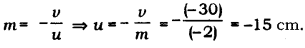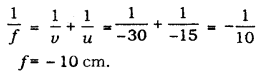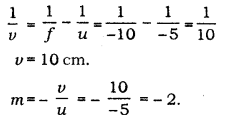# The image formed by a spherical mirror is real, inverted and is of magnification - 2

The image formed by a spherical mirror is real, inverted and is of magnification - 2. If the image is at a distance of 30 cm from the mirror, where is the object placed? Find the focal length of the mirror. List two characteristics of the image formed if the object is made at 10 cm towards the mirror.

Given:
Magnification, m = -2
Distance of the image, v = - 30 cm
Let u be the distance of the object from the mirror.
Since magnification of a mirror is given asThen, using the mirror formula, focal length (/) is found to beIf the image is moved to 10 cm towards the mirror, then the new position of the object is given as u’ = - (15 - 10) cm = - 5 cm
Then, new position of image is given bySince v is positive, the image will be virtual.
Hence, the new image will be virtual, erect and magnified.